# Sets Notes | Study GATE Computer Science Engineering(CSE) 2023 Mock Test Series - GATE

## GATE: Sets Notes | Study GATE Computer Science Engineering(CSE) 2023 Mock Test Series - GATE

The document Sets Notes | Study GATE Computer Science Engineering(CSE) 2023 Mock Test Series - GATE is a part of the GATE Course GATE Computer Science Engineering(CSE) 2023 Mock Test Series.
All you need of GATE at this link: GATE

## Set Theory | Introduction

Set is a unordered collection of objects, known as elements or members of the set.
An element ‘a’ belong to a set A can be written as ‘a ∈ A’,  ‘a ∉ A’ denotes that a is not an element of the set A.

Representation of a Set
A set can be represented by various methods. 3 common methods used for representing set:
1. Statement form.
2. Roaster form or tabular form method.
3. Set Builder method.

Statement form
In this representation, well defined description of the elements of the set is given. Below are some examples of same.
1. The set of all even number less than 10.
2. The set of number less than 10 and more than 1.

Roster form
In this representation, elements are listed within the pair of brackets {} and are separated by commas. Below are two examples.
1. Let N is the set of natural numbers less than 5.
N = { 1 , 2 , 3, 4 }.
2. The set of all vowels in english alphabet.
V = { a , e , i , o , u }.

Set builder form
In set builder set is describe by a property that its member must satisfy.
1. {x : x is even number divisible by 6 and less than 100}.
2. {x : x is natural number less than 10}.

Equal sets
Two sets are said to be equal if both have same elements. For example A = {1, 3, 9, 7} and B = {3, 1, 7, 9} are equal sets.

NOTE: Order of elements of a set doesn’t matter.

Subset
A set A is said to be subset of another set B if and only if every element of set A is also a part of other set B.
Denoted by ‘‘.
‘A ⊆ B ‘ denotes A is a subset of B.

To prove A is subset of B, we need to simply show that if x belongs to A then x also belongs to B.
To prove A is not a subset of B, we need find out one element which is part of set A but not belong to set B.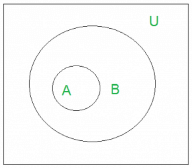‘U’ denotes the universal set.
Above Venn Diagram shows that A is Subset of B.

Size of a Set
Size of a set can be finite or infinite.

For example

Finite set: Set of natural numbers less than 100.
Infinite set: Set of real numbers.

Size of the set S is known as Cardinality number, denoted as |S|.

Example: Let A be a set of odd positive integers less than 10.
Solution : A = {1,3,5,7,9}, Cardinality of the set is 5, i.e.,|A| = 5.

Note: Cardinality of null set is 0.

Power Sets
Power set is the set all possible subset of the set S. Denoted by P(S).
Example : What is the power set of {0,1,2}?
Solution: All possible subsets
{∅}, {0}, {1}, {2}, {0,1}, {0,2}, {1,2}, {0,1,2}.
Note : Empty set and set itself is also member of this set of subsets.

Cardinality of power set is 2n, where n is number of element in a set.

Cartesian Products
Let A and B be two sets. Cartesian product of A and B is denoted by A × B, is the set of all ordered pairs (a,b), where a belong to A and B belong to B.

A × B = {(a, b) | a ∈ A ∧ b ∈ B}.

Example 1. What is Cartesian product of A = {1,2} and B = {p,q,r}.
Solution : A × B ={(1,p), (1,q), (1,r), (2,p), (2,q), (2,r) };

Cardinality of A × B  is N*M, where N is the Cardinality of A and M is the cardinality of B.

Note : A × B is not same as B × A.

## Set theory | Set Operations

Union
Union of the sets A and B, denoted by A ∪ B, is the set of distinct element belongs to set A or set B, or both.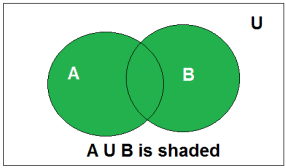Above is the Venn Diagram of A U B.

Example : Find the union of A = {2, 3, 4} and B = {3, 4, 5};
Solution : A ∪ B = {2, 3, 4, 5}.

Intersection
Intersection of the sets A and B, denoted by A ∩ B, is the set of elements belongs to both A and B i.e. set of common element in A and B.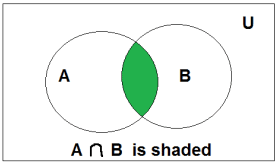Above is the Venn Diagram of A ∩ B.

Example: Consider the previous sets A and B. Find out A ∩ B.
Solution : A ∩ B = {3, 4}.

Disjoint
Two sets are said to be disjoint if their their intersection is the empty set .i.e sets have no common elements.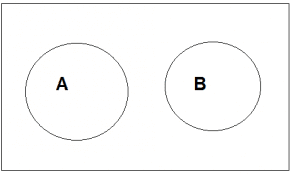Above is the Venn Diagram of A disjoint B.

For Example
Let A = {1, 3, 5, 7, 9} and B = { 2, 4 ,6 , 8} .
A and B are disjoint set both of them have no common elements.

Set Difference
Difference between sets is denoted by ‘A – B’ , is the set containing elements of set A but not in B. i.e all elements of A except the element of B.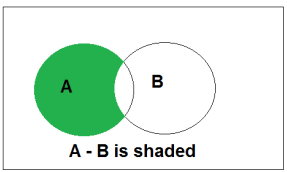Above is the Venn Diagram of A-B.

Complement
Complement of a set A, denoted by AC

Example : Let A = {0, 2, 4, 6, 8} , B = {0, 1, 2, 3, 4} and C = {0, 3, 6, 9}. What are A ∪ B ∪ C and A ∩ B ∩ C ?

Solution : Set A ∪ B ∪ C contains elements which are present in at least one of A, B and C.

A ∪ B ∪ C = {0, 1, 2, 3, 4, 6, 8, 9}.

Set A ∩ B ∩ C contains element which are present in all the sets A, B and C .i.e {0}.

The document Sets Notes | Study GATE Computer Science Engineering(CSE) 2023 Mock Test Series - GATE is a part of the GATE Course GATE Computer Science Engineering(CSE) 2023 Mock Test Series.
All you need of GATE at this link: GATEUse Code STAYHOME200 and get INR 200 additional OFF

## GATE Computer Science Engineering(CSE) 2023 Mock Test Series

136 docs|165 tests

Track your progress, build streaks, highlight & save important lessons and more!

,

,

,

,

,

,

,

,

,

,

,

,

,

,

,

,

,

,

,

,

,

;